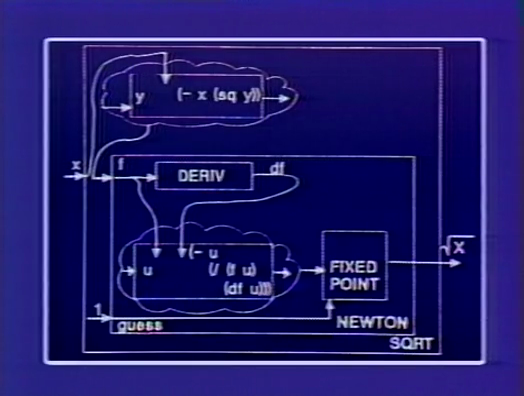# SICP 2a Higher-order Procedures

## 1 Déjà vu

$∑ a≤i≤b i$

(define (sum-int a b)
(if (> a b)
0
(+ a (sum-int (1+ a) b))))


$∑ a≤i≤b i2$

(define (sum-sq a b)
(if (> a b)
0
(+ (sq a) (sum-int (1+ a) b))))


Computers were created to take care of menial tasks, so avoid repitition. Divide everything into smaller pieces, then each small piece needs to be debugged/understood only once.

$∑ a≤i≤b by 4 1i(i + 2)$

(define (pi-sum a b)
(if (> a b)
0
(+ (/1 (* a (+ a 2)))
(pi-sum (+ a 4) b))))


## 2 Procedure as argument

When dealing with patterns, find the abstraction.

General pattern in the above sums:

(define (<name> a b)
(if (> a b)
0
(+ (<term> a)
(<name> (<next> a) b))))


And now more correctly:

(define (sum term a next b)
(if (> a b)
0
(+ (term a)
(sum term (next a) next b))))


term and next are both procedural arguments.

Rewritten sum-int, sum-sq and pi-sum are shown below.

(define (sum-int a b)
(define (identity a) a)
(sum identity a 1+ b))

(define (sum-sq a b)
(sum sq a 1+ b))

(define (pi-sum a b)
(sum (lambda (i) (/ 1 (* i (+ i 2))))
a
(lambda (i) (+ i 4))
b))


There are multiple ways of implementing sum. Below is an iterative version.

(define (sum term a next b)
(define (iter j ans)
(if (> j b)
ans
(iter (next j)
(+ (term j) ans))))
(iter a 0))


## 3 Procedure as value

Computers to make people happy, not people to make computers happy.

Returning to the square root procedure, note that we have been searching for a fixed point of $f$. ($x$ is a fixed point of $f$ if $f\left(x\right)=x$.)

$y ↦𝑓 y+ xy 2$

$f(x) = x$

(define (sqrt x)
(fixed-point
(lambda (y) (average (/x y) y))
1))

(define (fixed-point f start)
(define tolerance 0.00001)
(define (close-enough? u v)
(< (abs (- u v)) tolerance))
(define (iter old new)
(if (close-enough? old new)
new
(iter new (f new))))
(iter start (f start)))


Note that $y↦\frac{x}{y}$ with $y=1$ and $x=2$ leads to a never-ending oscillation. The dampener is required to ensure that progress towards to answer is maintained.

More clearly stated:

(define (sqrt x)
(fixed-point
(average-damp (lambda (y) (/ x y)))
1))
(define average-damp
(lambda (f)
(lambda (x) (average (f x) x)))


average-damp takes a procedure as an argument and returns a procedure as a value.

## 4 Newton's method

Newton's method, also known as the Newton-Raphson method, is an algorithm to produce successively better approximations to the zeroes (i.e. roots) of functions. (Find $y$ s.t. $f(y)=0$ .)

Start with a guess $y+0$ . Then

$y_{n+1}=y_{n}-\dfrac{f(y_{n})}{\frac{df}{dy}|_{y=y_{n}}}$

Re-implementing sqrt using Newton's method:

(define (sqrt x)
(newton (lambda (y) (- x (square y)))
1))

(define (newton f guess)
(define df (deriv f))
(fixed-point
(lambda (x) (- x (/ (f x) (df x))))
guess))


Use wishful thinking to assume magic can be done with anything with a name. Wishful thinking is essential for good computer science.

(define deriv
(lambda (f)
(lambda (x)
(/ (- (f (+ x dx))
(f x))
dx))))
(define dx 0.000001)


And magically we have the derivative! Evidently this isn't perfect and should make numerical analysts skin crawl.

## 5 A thousand words## 6 First-class citizens

Christopher Strachey, grandfather of computer science, logician and inventor of denotational semantics, was one of the first to make procedures first-class citizens in programming langauges.

The rights & privileges of first-class citizens

• To be named by variables.

• To be passed as arguments to procedures.

• To be returned as values of procedures.

• To be incorporated into data structures.

Turning procedures into first-class citizens allows for powerful abstractions.

Note to self: orgmode and latexml are lifesavers.